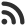Feed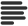Articles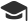Tutorials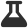Lab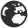CompaniesLeaderboard
 DG Student at BIT Mesra Oct. 12, 2020, 1:22 p.m. ⋅ 734 views

# TCS NQT Technical Questions set 1

1. In C, if you pass an array as an argument to a function, what actually gets passed?

A. Value of elements in array
B. First element of the array
C. Base Address of the array
D. Address of the last element of array

Ans. C

2. Are the expressions arr and &arr same for an array of 10 integers?

A. True
B. False

Ans. B

3. Where the local variables are stored?

A. Stack
B. Heap
C. Disk
D. None of these

Ans. A

4. Print the output of following code :

``````main()
{
if(-1)
printf(“True”);
else
printf(“False”);
}``````

A. True
B. False
C. True False
D. Error

Ans. A

5. Which of the following is a collection of different data types?

A. String
B. Structure
C. Array
D. None of these

Ans. B

6. Process in which source code is combined with object code is termed as :

D. None of these

Ans. C

7. For 32 bits environment, size of ‘int’ data type is :

A. 2 Bytes
B. 4 Bytes
C. 8 Bytes
D. None of these

Ans. B

8. In C, the names of variables, functions, labels, and various other user-defined items are :

A. Keywords
B. Tokens
C. Identifiers
D. None of these

Ans. C

9. Variables that are declared inside a function are :

A. Constants
B. Literals
C. Global
D. Local

Ans. D

10. What will happen if in a C program you assign a value to an array element whose subscript exceeds the size of array?

A. The element will be set to 0.
B. The compiler would report an error.
C. The program may crash if some important data gets overwritten.
D. The array size would appropriately grow.

Ans. C

11. What can not be declared :

A. Array of Integers
B. Array of Pointers
C. Array of Structures
D. None of these

Ans. D

12. Which of the following is not a arithmetic operation :

A. n/=10
B. n*=10
C. n+=10
D. n!=10

Ans. D

13. Which datatype is not applicable for mod(%) operation ?

A. int
B. float
C. char
D. None of these

Ans. D

14. Strings are character arrays. The last index of it contains the null-terminated character :

A. \t
B. \n
C. \0
D. \o

Ans. C

15. ______ is the collection of similar-type elements ?

A. Structure
B. Union
C. Array
D. String

Ans. C

16. Which has the highest precision ?

A. float
B. double
C. long int
D. unsigned long int

Ans. B

17. Which of the following is a user-defined data type ?

A. long int
B. double
C. unsigned long int
D. enum

Ans. D

18. Which of the following are Abstract Datatypes ?

A. int
B. structure
C. char
D. float

Ans. B

19. Which of the following is for releasing memory by dynamic memory allocation ?

A. release
B. revoke
C. allod
D. free

Ans. D

20. Which of the following is a collection of different data types?

A. String
B. Structure
C. Array
D. Files

Ans. B

21. int **p; What does this mean ?

A. pointer to int
B. pointer to pointer
C. error
D. None of these

Ans. B

22. Variables can be started with :

A. !
B. \$
C. _
D. .

Ans. C

23. All keywords in C are written in :

A. upper case
B. lower case
C. camel case
D. None of the above

Ans. B

24. Which keyword is responsible for skipping the current iteration ?

A. break
B. continue
C. return
D. pass

Ans. B

25. In the standard library of C programming language, which of the following header file is designed for basic mathematical operations?

A. conio.h
B. stdlib. h
C. math.h
D. calc.h

Ans. C

26. In C, compiler generally ignores the statement starting with :

A. //
B. /
C. */
D. None of these

Ans. A

27. A type of pointer which has not been initialized :

A. dangling pointer
B. default pointer
C. wild pointer
D. None of these

Ans. C

28. The main purpose of ‘ ftell ‘ :

A. getting current file name
B. getting current file status
C. getting current file attributes
D. getting current file position

Ans. D

29. Where are the local variables are stored ?

A. Disk
B. Stack
C. Heap
D. OS

Ans. B

30. What is the use of \r in C?

A. used to insert a vertical tab
B. used to insert a tab
C. places cursor at the end of line
D. places cursor at the start of line

Ans. D

Events

Nov. 28, 2018, 5:30 p.m.

Python from zero to hero

place Delhi

Aug. 13, 2018, 5:30 p.m.

Python from zero to hero

place Bangalore ( HackersFriend office BTM Layout)